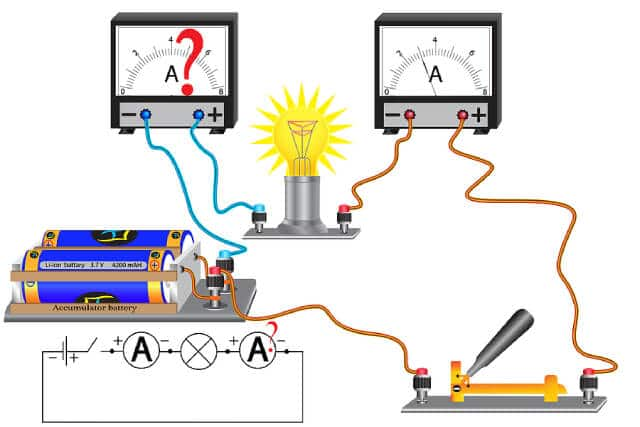The study of electric current is more than just about understanding what electricity is, but how it travels from one place to the next! Have you ever wondered how electricity plays a vital part in our lives, especially in many different real-world applications? This is where the study of electrical current, such as conventional current and electron flow, can help us process what is happening in a circuit.

Here’s what you need to know about the definitions and measurements of electrical current, conventional current, electron flow and more!

Understanding current and charges

Electric current is about the movement of electrical charges. It refers to total amount of charge that passes through a wire in a period of time. For electric current to pass around a circuit, there needs to be a potential difference between two points – and this is often created by a source like a battery. The potential difference per unit charge is what we call voltage.

The current is generated by charge carriers, which are particles such as electrons and ions that carry these electric charges around the component.

Conventional current & electron flow

The charge carriers in a metal conductor are electrons. Conventional current describes the movement of positive charges, which goes from the positive terminal of the source, to the negative terminal. This is the reverse of electron flow. As electrons are negatively charged, electron flow goes in the direction from the negative terminal to the positive terminal.

Measurement units for electric current

The measurement unit of charge is Coulomb (C).

The measurement unit for current is Amperes (A). 1A is defined as the current flow of 1C of charge in 1 second.

The relationship of charge and current in their respective units can be described by the formula as follows:

(I – electric current (A), Q – the rate of flow of charges (C), t – time (s))

Examples of calculating charge flow in a circuit

• 1A in 5 seconds is 5C
• 5A in 100 seconds is 500C
• 10mA in 500 seconds is 5C

Test for conduction

Sometimes, we might want to find out how well a material can conduct electricity. We can use a tool for measuring current, called an ammeter. A simple test for electrical conduction would involve an ammeter, a test material, and a battery. It is connected and linked by wires into a closed circuit. The current would pass from the cell, to the conducting material, through the ammeter, and finally returning back to the battery. If the material is a conductor, the ammeter will show a reading of the current that passes through.

Here is an example of a simple test for electrical conduction:Electromotive force

Despite its name, electromotive force (e.m.f.) is not actually a force, but rather, a measure of the work done by a source in driving the unit charge around a complete circuit. Typically, it refers to the energy transferred from chemical energy to electrical energy at the battery. The SI unit for e.m.f is Volt (V).

To calculate e.m.f. when sources are placed in a series, this is the formula used:

E = W / Q

(E – e.m.f (V), W – work done by source (J), Q – the amount of positive charges (C))

Potential difference

Not to be confused with the potential difference between two points in a circuit, potential difference (p.d.) of a component in a circuit is the measurement of the work done in driving the unit charge through the circuit component. It usually refers to energy transfer from electrical energy to other forms, for example, heat or light in a bulb. The SI unit for p.d. is Volt (V).

To calculate the potential difference, the formula used is:

V = W / Q

(V – potential difference (V), W – work done to drive the charge between two points (J), Q – the total amount of positive charges (C))

Essentially, you can say that e.m.f. and p.d. of a component uses the same formula. But they describe different phenomena occurring at different points of the circuit.

It is important to note that the electromotive force can occur without a closed circuit. However, there cannot be a potential difference without a closed circuit.

Conclusion

There is so much more to learn about electric currents, and if you are ready to learn, then the best way to do so is to enrol in a physics tuition class! It can be challenging to learn new physics topics on your own. With a physics tutor to guide you, you can learn more about important definitions, get examples, and improve your physics learning!

Not only can you speak to your tutor about all of the problems that you might face while learning electric currents, but you’ll be able to receive the guidance you need to conquer the physics topics that are challenging you!